# RD Sharma Solutions for Class 11 Chapter 16 - Permutations Exercise 16.2

This exercise deals with two fundamental principles (i) principle of addition and (ii) principle of multiplication. These two principles will enable us to understand the concept of permutations and combinations. This exercise contains various solved examples before the exercise wise problems start, in order to help students practice numerous problems to obtain a better academic score. The solutions are formulated by teachers after conducting vast research on each concept so that students improve their logical approach and their abilities to analyse the type of questions that would appear in the board exam. For a better conceptual idea, students can access RD Sharma Class 11 Maths Solutions free pdf, from the links, which are provided below.

## Download the pdf of RD Sharma Solutions for Class 11 Maths Exercise 16.2 Chapter 16 – Permutations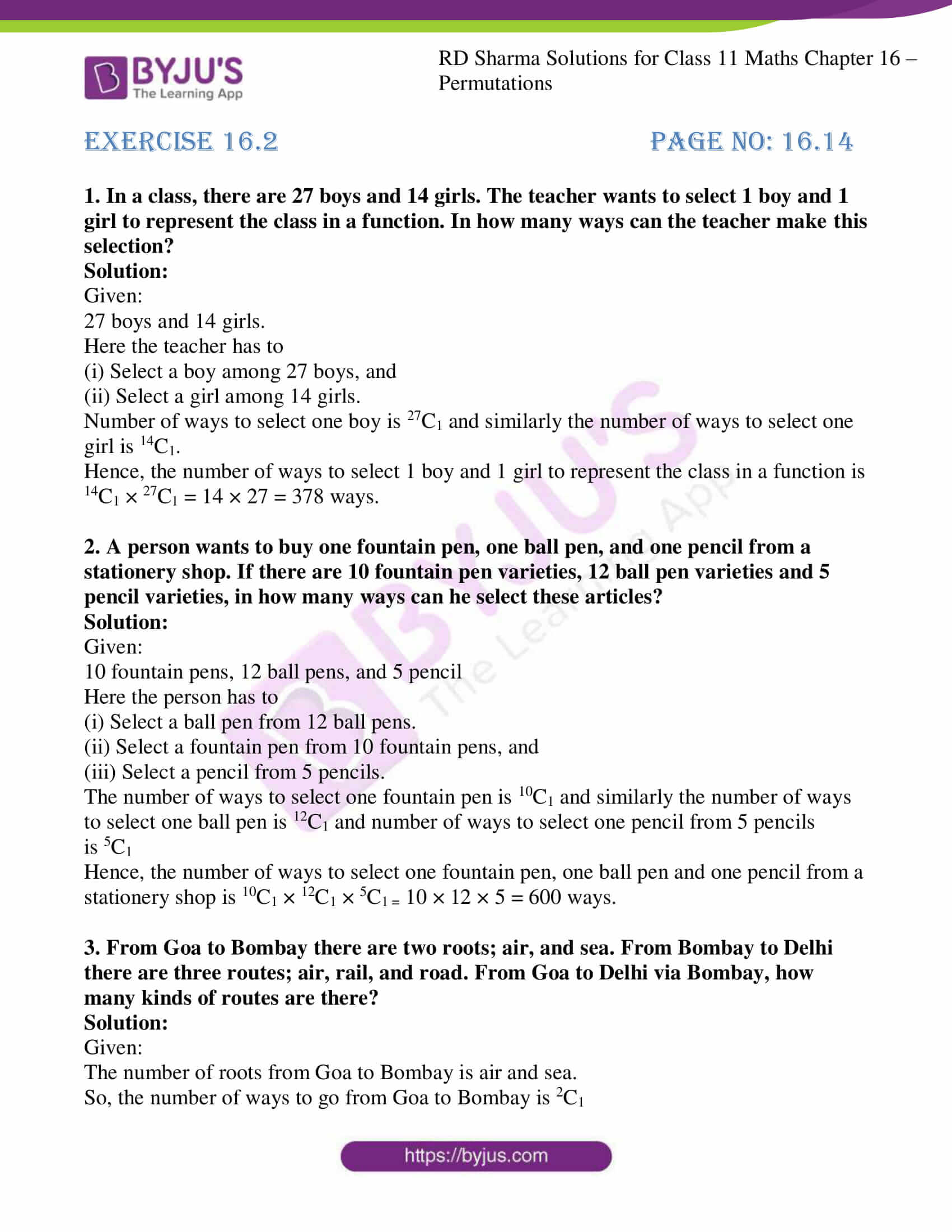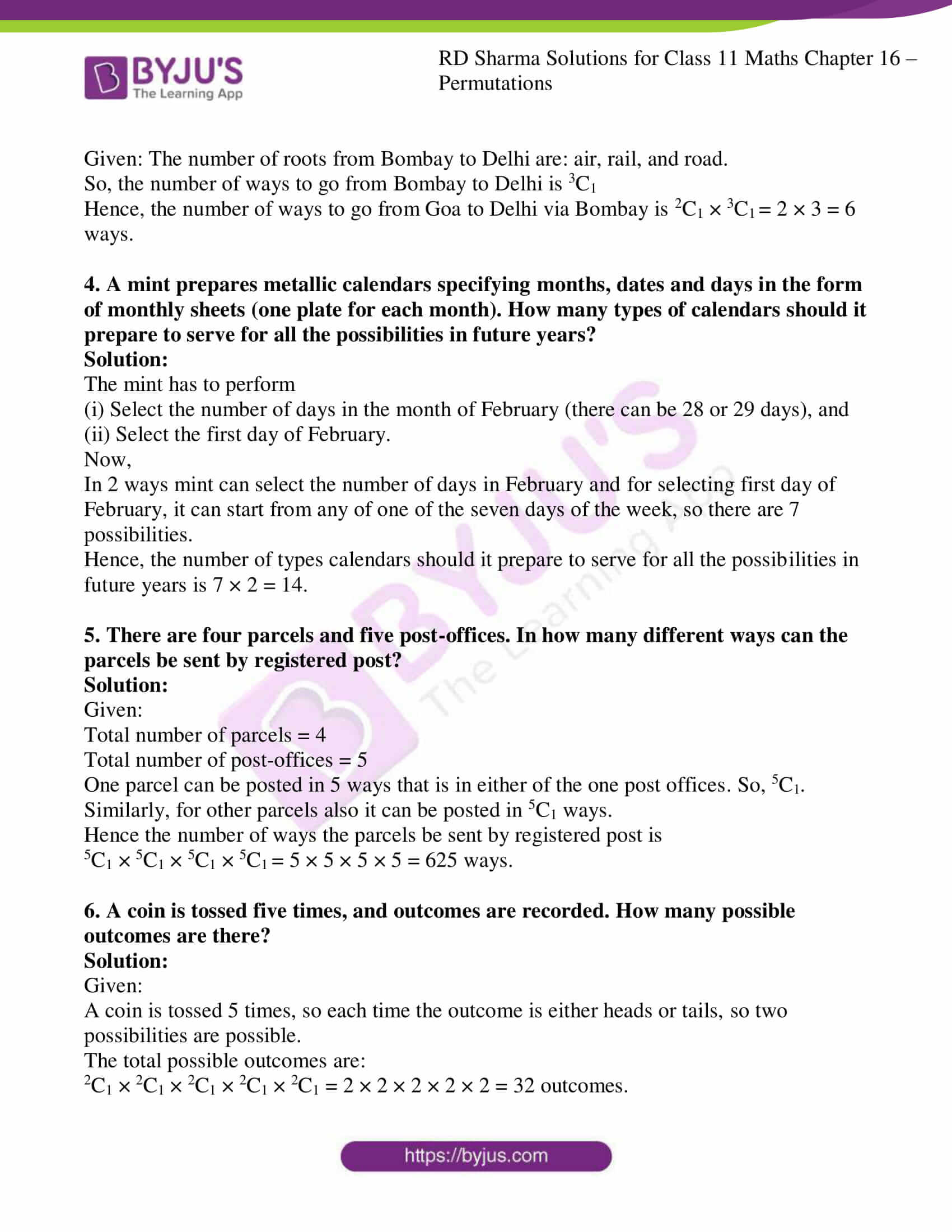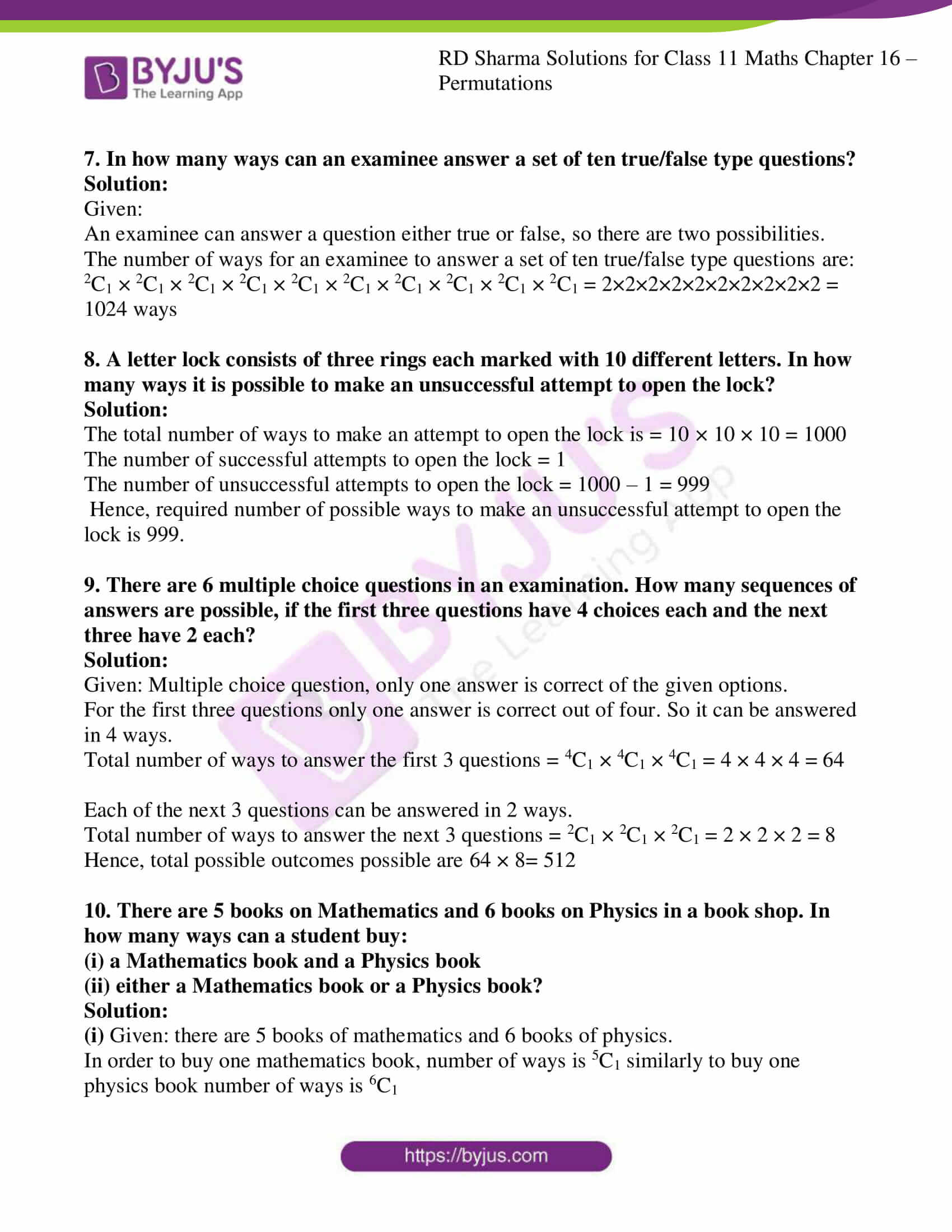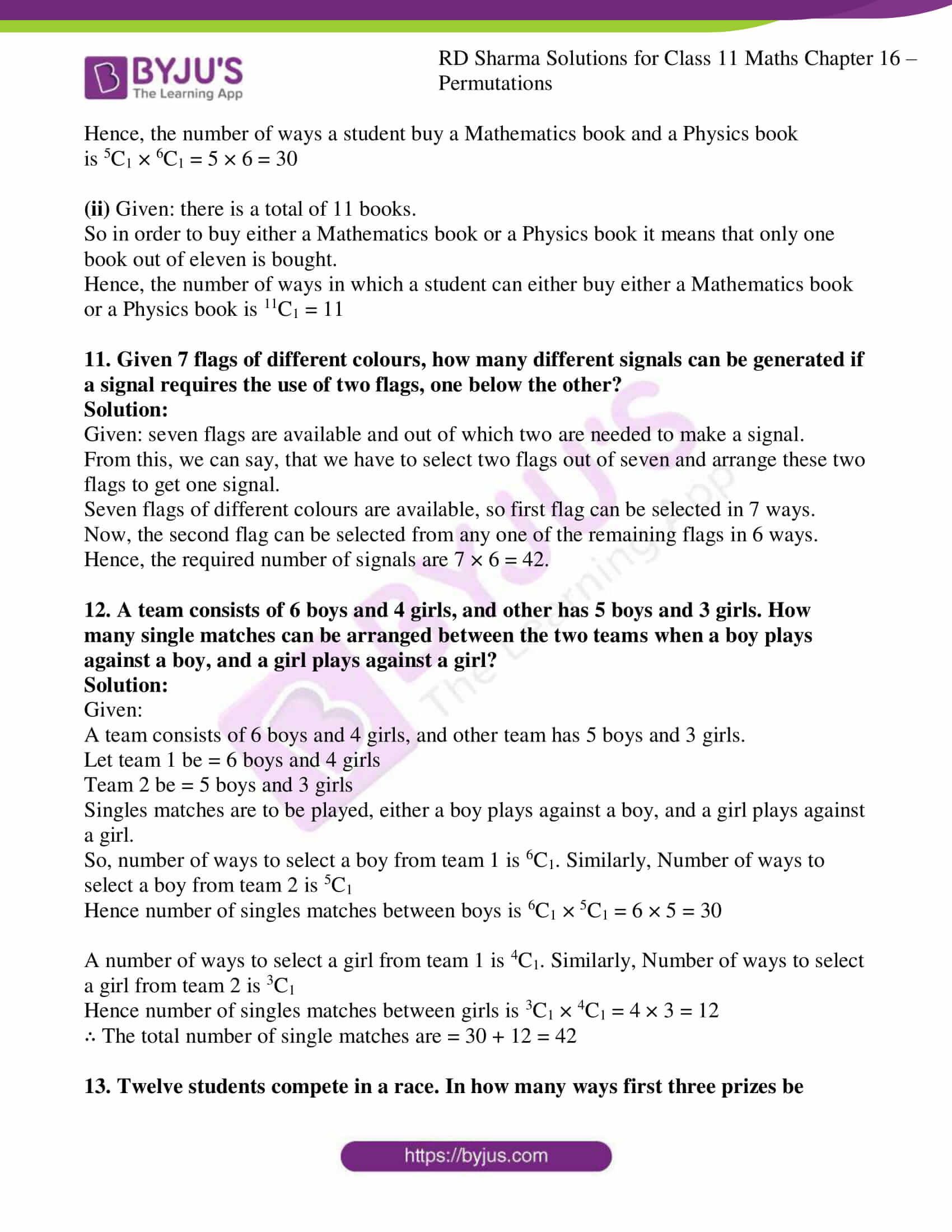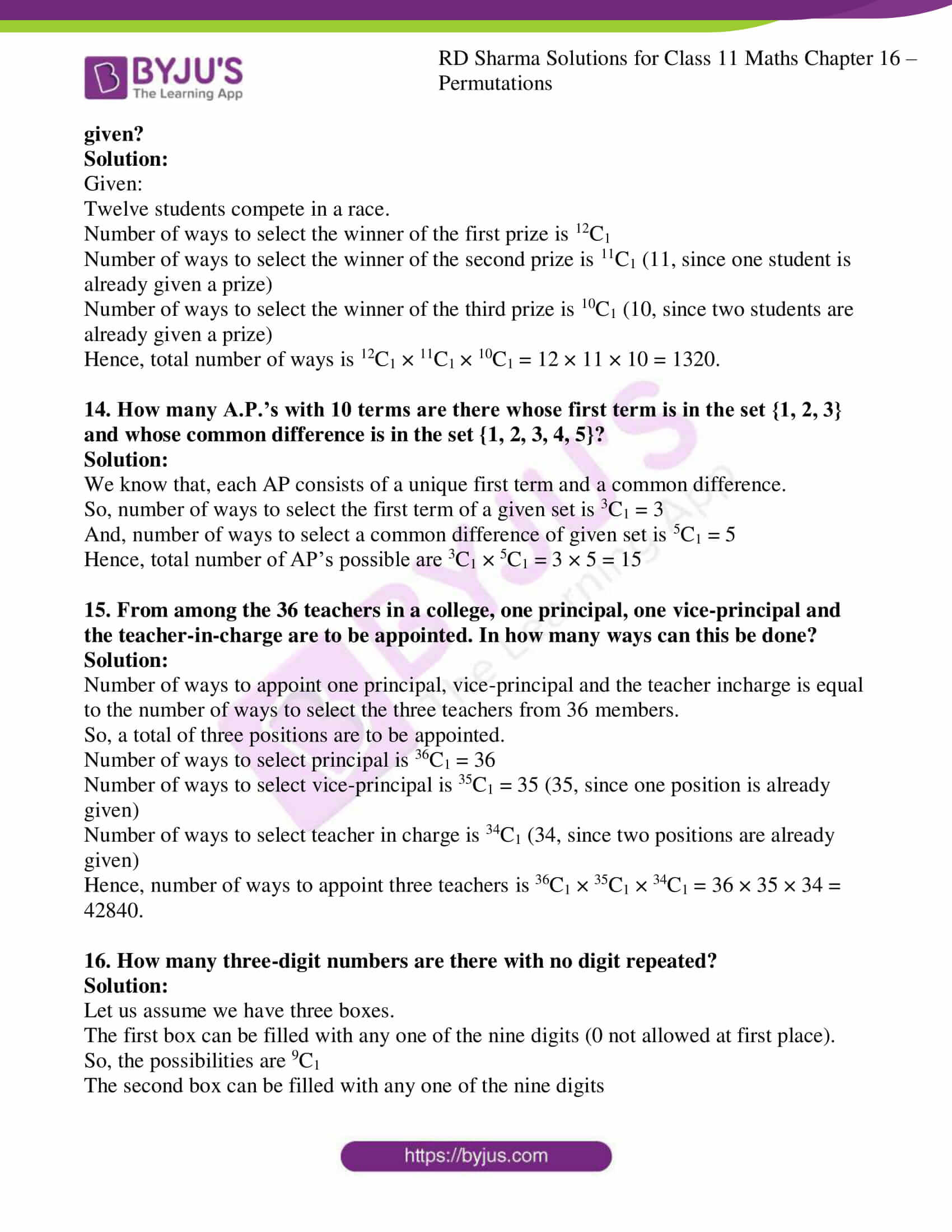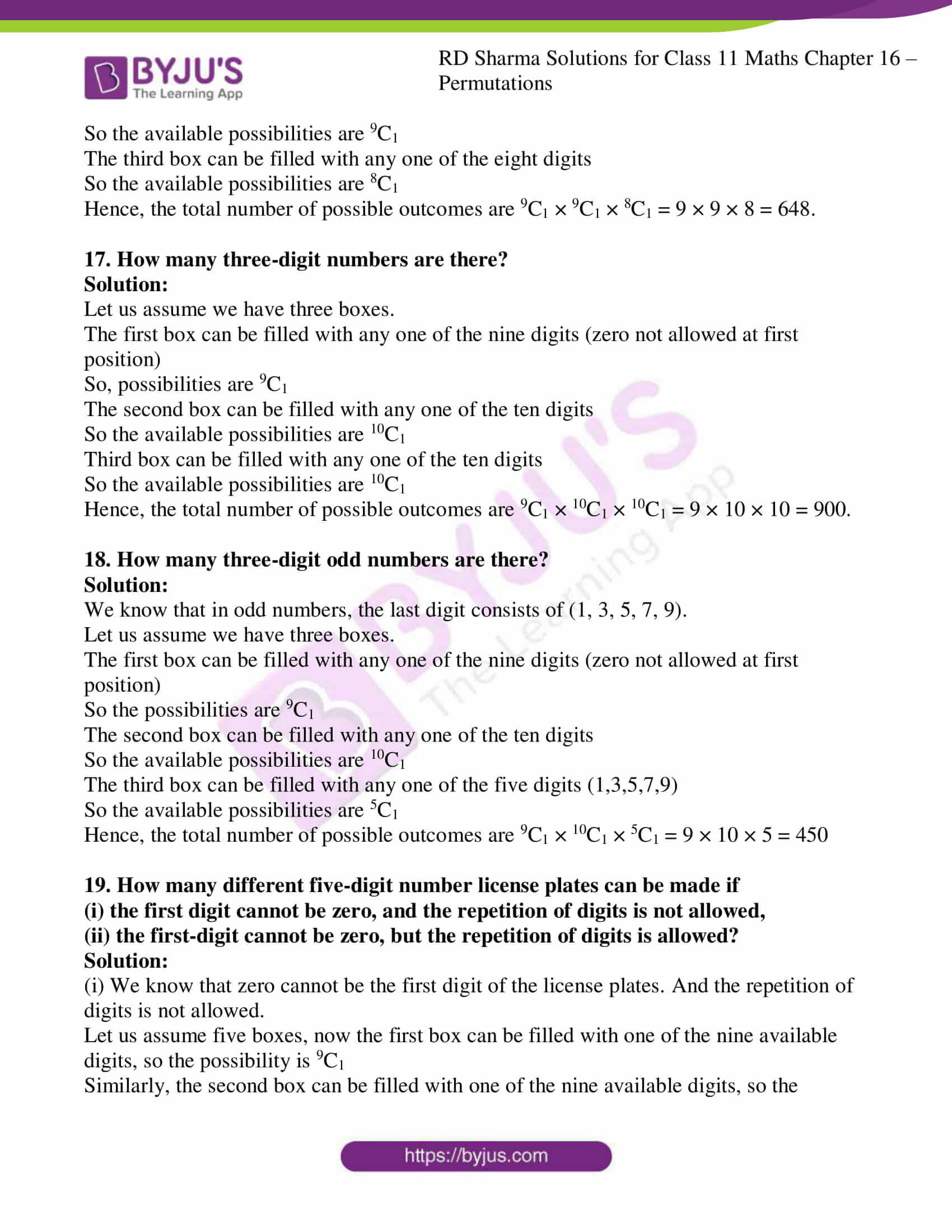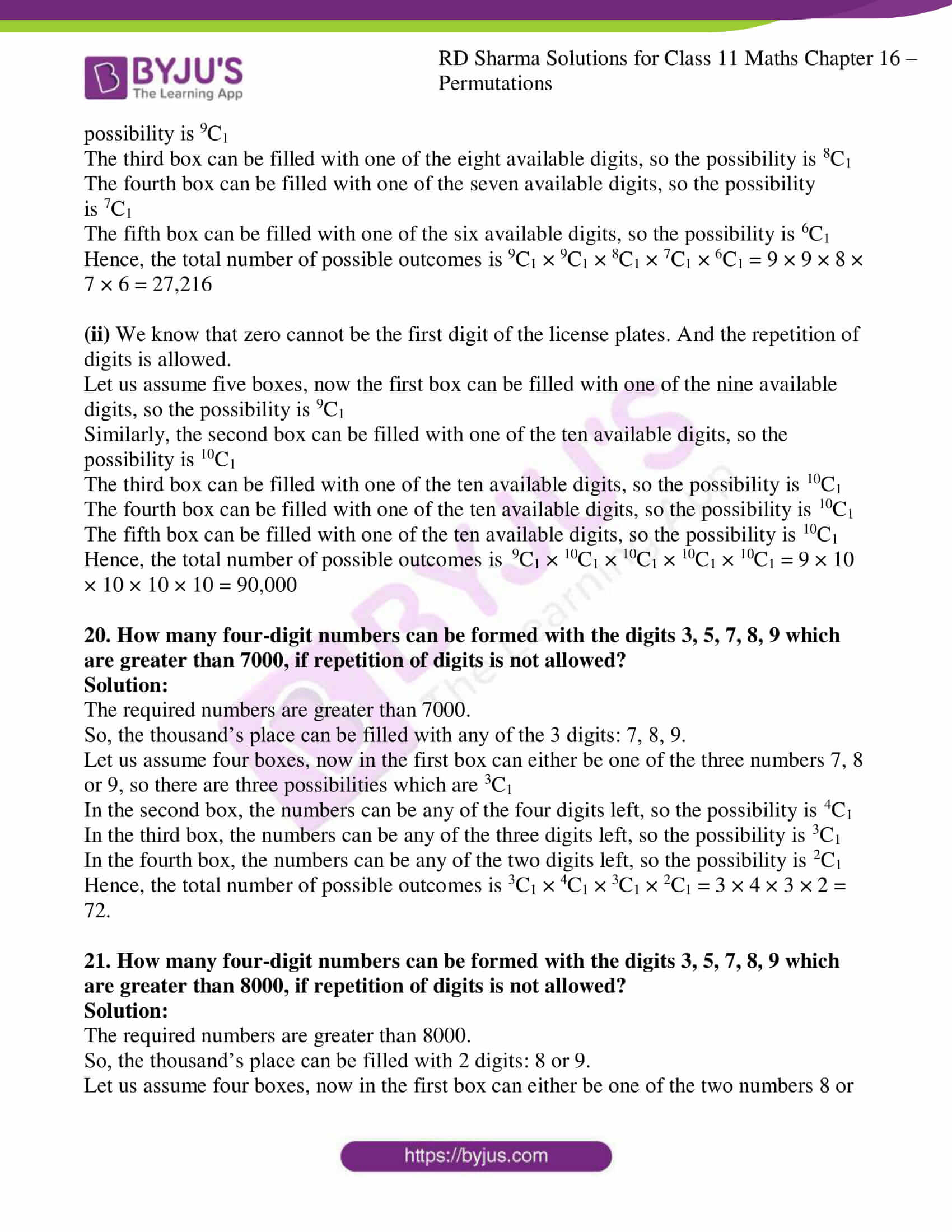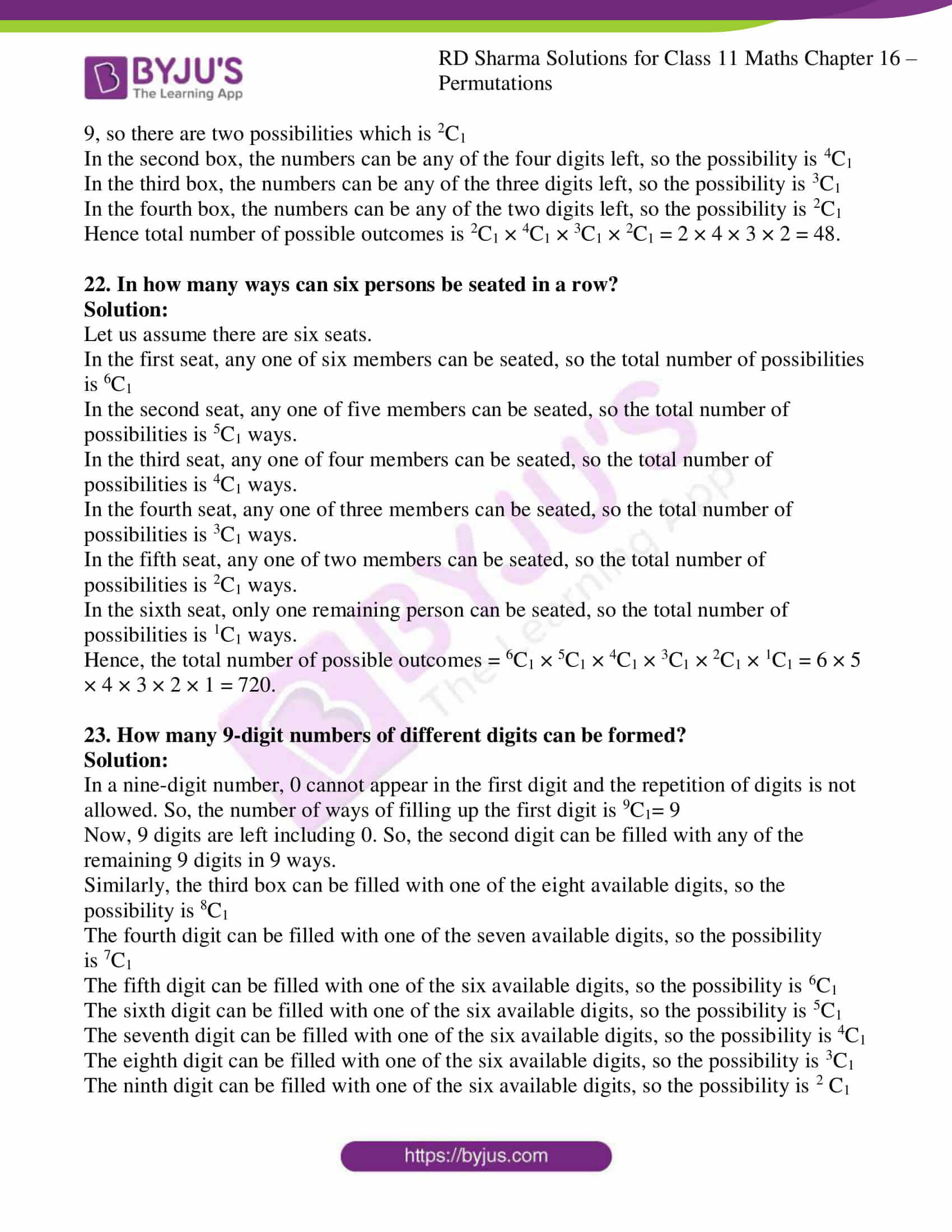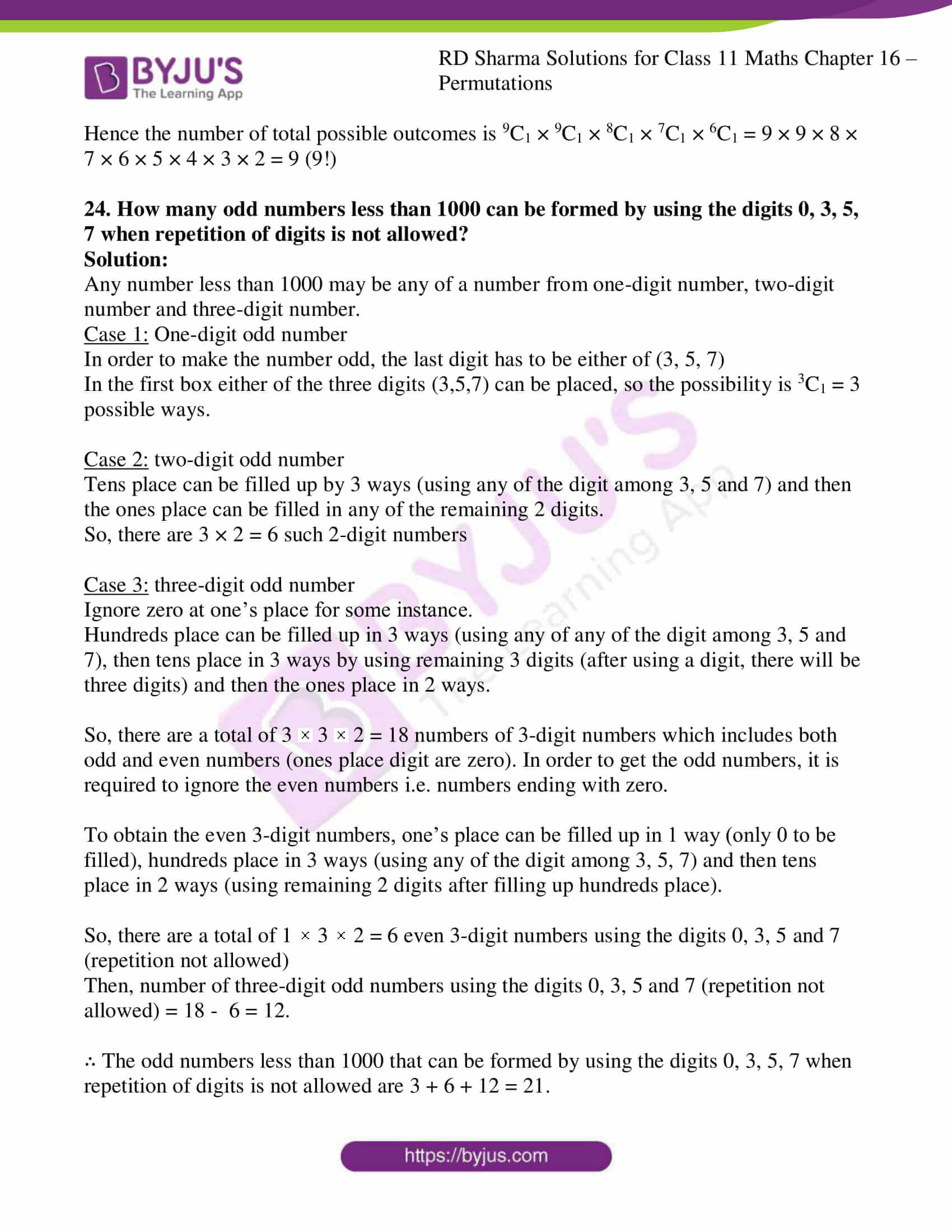### Access answers to RD Sharma Solutions for Class 11 Maths Exercise 16.2 Chapter 16 – Permutations

1. In a class, there are 27 boys and 14 girls. The teacher wants to select 1 boy and 1 girl to represent the class in a function. In how many ways can the teacher make this selection?

Solution:

Given:

27 boys and 14 girls.

Here the teacher has to

(i) Select a boy among 27 boys, and

(ii) Select a girl among 14 girls.

Number of ways to select one boy is 27C1 and similarly the number of ways to select one girl is 14C1.

Hence, the number of ways to select 1 boy and 1 girl to represent the class in a function is

14C1 × 27C1 = 14 × 27 = 378 ways.

2. A person wants to buy one fountain pen, one ball pen, and one pencil from a stationery shop. If there are 10 fountain pen varieties, 12 ball pen varieties and 5 pencil varieties, in how many ways can he select these articles?

Solution:

Given:

10 fountain pens, 12 ball pens, and 5 pencil

Here the person has to

(i) Select a ball pen from 12 ball pens.

(ii) Select a fountain pen from 10 fountain pens, and

(iii) Select a pencil from 5 pencils.

The number of ways to select one fountain pen is 10C1 and similarly the number of ways to select one ball pen is 12C1 and number of ways to select one pencil from 5 pencils is 5C1

Hence, the number of ways to select one fountain pen, one ball pen and one pencil from a stationery shop is 10C1 × 12C1 × 5C1 = 10 × 12 × 5 = 600 ways.

3. From Goa to Bombay there are two roots; air, and sea. From Bombay to Delhi there are three routes; air, rail, and road. From Goa to Delhi via Bombay, how many kinds of routes are there?

Solution:

Given:

The number of roots from Goa to Bombay is air and sea.

So, the number of ways to go from Goa to Bombay is 2C1

Given: The number of roots from Bombay to Delhi are: air, rail, and road.

So, the number of ways to go from Bombay to Delhi is 3C1

Hence, the number of ways to go from Goa to Delhi via Bombay is 2C1 × 3C1 = 2 × 3 = 6 ways.

4. A mint prepares metallic calendars specifying months, dates and days in the form of monthly sheets (one plate for each month). How many types of calendars should it prepare to serve for all the possibilities in future years?

Solution:

The mint has to perform

(i) Select the number of days in the month of February (there can be 28 or 29 days), and

(ii) Select the first day of February.

Now,

In 2 ways mint can select the number of days in February and for selecting first day of February, it can start from any of one of the seven days of the week, so there are 7 possibilities.

Hence, the number of types calendars should it prepare to serve for all the possibilities in future years is 7 × 2 = 14.

5. There are four parcels and five post-offices. In how many different ways can the parcels be sent by registered post?

Solution:

Given:

Total number of parcels = 4

Total number of post-offices = 5

One parcel can be posted in 5 ways that is in either of the one post offices. So, 5C1. Similarly, for other parcels also it can be posted in 5C1 ways.

Hence the number of ways the parcels be sent by registered post is

5C1 × 5C1 × 5C1 × 5C1 = 5 × 5 × 5 × 5 = 625 ways.

6. A coin is tossed five times, and outcomes are recorded. How many possible outcomes are there?

Solution:

Given:

A coin is tossed 5 times, so each time the outcome is either heads or tails, so two possibilities are possible.

The total possible outcomes are:

2C1 × 2C1 × 2C1 × 2C1 × 2C1 = 2 × 2 × 2 × 2 × 2 = 32 outcomes.

7. In how many ways can an examinee answer a set of ten true/false type questions?

Solution:

Given:

An examinee can answer a question either true or false, so there are two possibilities.

The number of ways for an examinee to answer a set of ten true/false type questions are: 2C1 × 2C1 × 2C1 × 2C1 × 2C1 × 2C1 × 2C1 × 2C1 × 2C1 × 2C1 = 2×2×2×2×2×2×2×2×2×2 = 1024 ways

8. A letter lock consists of three rings each marked with 10 different letters. In how many ways it is possible to make an unsuccessful attempt to open the lock?

Solution:

The total number of ways to make an attempt to open the lock is = 10 × 10 × 10 = 1000

The number of successful attempts to open the lock = 1

The number of unsuccessful attempts to open the lock = 1000 – 1 = 999

Hence, required number of possible ways to make an unsuccessful attempt to open the lock is 999.

9. There are 6 multiple choice questions in an examination. How many sequences of answers are possible, if the first three questions have 4 choices each and the next three have 2 each?

Solution:

Given: Multiple choice question, only one answer is correct of the given options.

For the first three questions only one answer is correct out of four. So it can be answered in 4 ways.

Total number of ways to answer the first 3 questions = 4C1 × 4C1 × 4C1 = 4 × 4 × 4 = 64

Each of the next 3 questions can be answered in 2 ways.

Total number of ways to answer the next 3 questions = 2C1 × 2C1 × 2C1 = 2 × 2 × 2 = 8

Hence, total possible outcomes possible are 64 × 8= 512

10. There are 5 books on Mathematics and 6 books on Physics in a book shop. In how many ways can a student buy:
(i) a Mathematics book and a Physics book
(ii) either a Mathematics book or a Physics book?

Solution:

(i) Given: there are 5 books of mathematics and 6 books of physics.

In order to buy one mathematics book, number of ways is 5C1 similarly to buy one physics book number of ways is 6C1

Hence, the number of ways a student buy a Mathematics book and a Physics book is 5C1 × 6C1 = 5 × 6 = 30

(ii) Given: there is a total of 11 books.

So in order to buy either a Mathematics book or a Physics book it means that only one book out of eleven is bought.

Hence, the number of ways in which a student can either buy either a Mathematics book or a Physics book is 11C1 = 11

11. Given 7 flags of different colours, how many different signals can be generated if a signal requires the use of two flags, one below the other?

Solution:

Given: seven flags are available and out of which two are needed to make a signal.

From this, we can say, that we have to select two flags out of seven and arrange these two flags to get one signal.

Seven flags of different colours are available, so first flag can be selected in 7 ways.

Now, the second flag can be selected from any one of the remaining flags in 6 ways.

Hence, the required number of signals are 7 × 6 = 42.

12. A team consists of 6 boys and 4 girls, and other has 5 boys and 3 girls. How many single matches can be arranged between the two teams when a boy plays against a boy, and a girl plays against a girl?

Solution:

Given:

A team consists of 6 boys and 4 girls, and other team has 5 boys and 3 girls.

Let team 1 be = 6 boys and 4 girls

Team 2 be = 5 boys and 3 girls

Singles matches are to be played, either a boy plays against a boy, and a girl plays against a girl.

So, number of ways to select a boy from team 1 is 6C1. Similarly, Number of ways to select a boy from team 2 is 5C1

Hence number of singles matches between boys is 6C1 × 5C1 = 6 × 5 = 30

A number of ways to select a girl from team 1 is 4C1. Similarly, Number of ways to select a girl from team 2 is 3C1

Hence number of singles matches between girls is 3C1 × 4C1 = 4 × 3 = 12

∴ The total number of single matches are = 30 + 12 = 42

13. Twelve students compete in a race. In how many ways first three prizes be given?

Solution:

Given:

Twelve students compete in a race.

Number of ways to select the winner of the first prize is 12C1

Number of ways to select the winner of the second prize is 11C1 (11, since one student is already given a prize)

Number of ways to select the winner of the third prize is 10C1 (10, since two students are already given a prize)

Hence, total number of ways is 12C1 × 11C1 × 10C1 = 12 × 11 × 10 = 1320.

14. How many A.P.’s with 10 terms are there whose first term is in the set {1, 2, 3} and whose common difference is in the set {1, 2, 3, 4, 5}?

Solution:

We know that, each AP consists of a unique first term and a common difference.

So, number of ways to select the first term of a given set is 3C1 = 3

And, number of ways to select a common difference of given set is 5C1 = 5

Hence, total number of AP’s possible are 3C1 × 5C1 = 3 × 5 = 15

15. From among the 36 teachers in a college, one principal, one vice-principal and the teacher-in-charge are to be appointed. In how many ways can this be done?

Solution:

Number of ways to appoint one principal, vice-principal and the teacher incharge is equal to the number of ways to select the three teachers from 36 members.

So, a total of three positions are to be appointed.

Number of ways to select principal is 36C1 = 36

Number of ways to select vice-principal is 35C1 = 35 (35, since one position is already given)

Number of ways to select teacher in charge is 34C1 (34, since two positions are already given)

Hence, number of ways to appoint three teachers is 36C1 × 35C1 × 34C1 = 36 × 35 × 34 = 42840.

16. How many three-digit numbers are there with no digit repeated?

Solution:

Let us assume we have three boxes.

The first box can be filled with any one of the nine digits (0 not allowed at first place).

So, the possibilities are 9C1

The second box can be filled with any one of the nine digits

So the available possibilities are 9C1

The third box can be filled with any one of the eight digits

So the available possibilities are 8C1

Hence, the total number of possible outcomes are 9C1 × 9C1 × 8C1 = 9 × 9 × 8 = 648.

17. How many three-digit numbers are there?

Solution:

Let us assume we have three boxes.

The first box can be filled with any one of the nine digits (zero not allowed at first position)

So, possibilities are 9C1

The second box can be filled with any one of the ten digits

So the available possibilities are 10C1

Third box can be filled with any one of the ten digits

So the available possibilities are 10C1

Hence, the total number of possible outcomes are 9C1 × 10C1 × 10C1 = 9 × 10 × 10 = 900.

18. How many three-digit odd numbers are there?

Solution:

We know that in odd numbers, the last digit consists of (1, 3, 5, 7, 9).

Let us assume we have three boxes.

The first box can be filled with any one of the nine digits (zero not allowed at first position)

So the possibilities are 9C1

The second box can be filled with any one of the ten digits

So the available possibilities are 10C1

The third box can be filled with any one of the five digits (1,3,5,7,9)

So the available possibilities are 5C1

Hence, the total number of possible outcomes are 9C1 × 10C1 × 5C1 = 9 × 10 × 5 = 450

19. How many different five-digit number license plates can be made if
(i) the first digit cannot be zero, and the repetition of digits is not allowed,
(ii) the first-digit cannot be zero, but the repetition of digits is allowed?

Solution:

(i) We know that zero cannot be the first digit of the license plates. And the repetition of digits is not allowed.

Let us assume five boxes, now the first box can be filled with one of the nine available digits, so the possibility is 9C1

Similarly, the second box can be filled with one of the nine available digits, so the possibility is 9C1

The third box can be filled with one of the eight available digits, so the possibility is 8C1

The fourth box can be filled with one of the seven available digits, so the possibility is 7C1

The fifth box can be filled with one of the six available digits, so the possibility is 6C1

Hence, the total number of possible outcomes is 9C1 × 9C1 × 8C1 × 7C1 × 6C1 = 9 × 9 × 8 × 7 × 6 = 27,216

(ii) We know that zero cannot be the first digit of the license plates. And the repetition of digits is allowed.

Let us assume five boxes, now the first box can be filled with one of the nine available digits, so the possibility is 9C1

Similarly, the second box can be filled with one of the ten available digits, so the possibility is 10C1

The third box can be filled with one of the ten available digits, so the possibility is 10C1

The fourth box can be filled with one of the ten available digits, so the possibility is 10C1

The fifth box can be filled with one of the ten available digits, so the possibility is 10C1

Hence, the total number of possible outcomes is  9C1 × 10C1 × 10C1 × 10C1 × 10C1 = 9 × 10 × 10 × 10 × 10 = 90,000

20. How many four-digit numbers can be formed with the digits 3, 5, 7, 8, 9 which are greater than 7000, if repetition of digits is not allowed?

Solution:

The required numbers are greater than 7000.

So, the thousand’s place can be filled with any of the 3 digits: 7, 8, 9.

Let us assume four boxes, now in the first box can either be one of the three numbers 7, 8 or 9, so there are three possibilities which are 3C1

In the second box, the numbers can be any of the four digits left, so the possibility is 4C1

In the third box, the numbers can be any of the three digits left, so the possibility is 3C1

In the fourth box, the numbers can be any of the two digits left, so the possibility is 2C1

Hence, the total number of possible outcomes is 3C1 × 4C1 × 3C1 × 2C1 = 3 × 4 × 3 × 2 = 72.

21. How many four-digit numbers can be formed with the digits 3, 5, 7, 8, 9 which are greater than 8000, if repetition of digits is not allowed?

Solution:

The required numbers are greater than 8000.

So, the thousand’s place can be filled with 2 digits: 8 or 9.

Let us assume four boxes, now in the first box can either be one of the two numbers 8 or 9, so there are two possibilities which is 2C1

In the second box, the numbers can be any of the four digits left, so the possibility is 4C1

In the third box, the numbers can be any of the three digits left, so the possibility is 3C1

In the fourth box, the numbers can be any of the two digits left, so the possibility is 2C1

Hence total number of possible outcomes is 2C1 × 4C1 × 3C1 × 2C1 = 2 × 4 × 3 × 2 = 48.

22. In how many ways can six persons be seated in a row?

Solution:

Let us assume there are six seats.

In the first seat, any one of six members can be seated, so the total number of possibilities is 6C1

In the second seat, any one of five members can be seated, so the total number of possibilities is 5C1 ways.

In the third seat, any one of four members can be seated, so the total number of possibilities is 4C1 ways.

In the fourth seat, any one of three members can be seated, so the total number of possibilities is 3C1 ways.

In the fifth seat, any one of two members can be seated, so the total number of possibilities is 2C1 ways.

In the sixth seat, only one remaining person can be seated, so the total number of possibilities is 1C1 ways.

Hence, the total number of possible outcomes = 6C1 × 5C1 × 4C1 × 3C1 × 2C1 × 1C1 = 6 × 5 × 4 × 3 × 2 × 1 = 720.

23. How many 9-digit numbers of different digits can be formed?

Solution:

In a nine-digit number, 0 cannot appear in the first digit and the repetition of digits is not allowed. So, the number of ways of filling up the first digit is 9C1= 9

Now, 9 digits are left including 0. So, the second digit can be filled with any of the remaining 9 digits in 9 ways.

Similarly, the third box can be filled with one of the eight available digits, so the possibility is 8C1

The fourth digit can be filled with one of the seven available digits, so the possibility is 7C1

The fifth digit can be filled with one of the six available digits, so the possibility is 6C1

The sixth digit can be filled with one of the six available digits, so the possibility is 5C1

The seventh digit can be filled with one of the six available digits, so the possibility is 4C1

The eighth digit can be filled with one of the six available digits, so the possibility is 3C1

The ninth digit can be filled with one of the six available digits, so the possibility is 2 C1

Hence the number of total possible outcomes is 9C1 × 9C1 × 8C1 × 7C1 × 6C1 = 9 × 9 × 8 × 7 × 6 × 5 × 4 × 3 × 2 = 9 (9!)